Type a math problemalgebra
trigonometry
statistics
calculus
matrices
variables
list
AC
log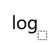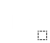ln
(
)
7
8
9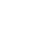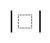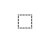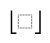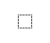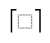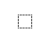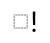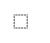τ
π
4
5
6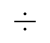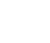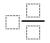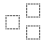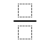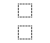%
θ
1
2
3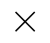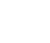<
>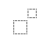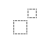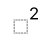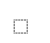x
i
0
.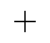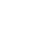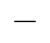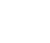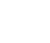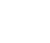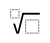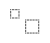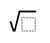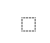y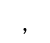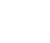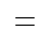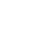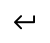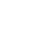Solve for x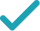Steps Using Factoring
Steps Using Factoring By Grouping
Steps for Completing the Square
Steps Using Direct Factoring Method
Steps Using FactoringTo solve the equation, factor using formula . To find and , set up a system to be solved.
Since is negative, and have the opposite signs. Since is negative, the negative number has greater absolute value than the positive. The only such pair is the system solution.
Rewrite factored expression using the obtained values.
To find equation solutions, solve and .
View solution steps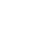GraphGraph Both Sides in 2D
Graph in 2D

## Similar Problems from Web Search

a+b=-4 ab=-5
To solve the equation, factor x^{2}-4x-5 using formula x^{2}+\left(a+b\right)x+ab=\left(x+a\right)\left(x+b\right). To find a and b, set up a system to be solved.
a=-5 b=1
Since ab is negative, a and b have the opposite signs. Since a+b is negative, the negative number has greater absolute value than the positive. The only such pair is the system solution.
\left(x-5\right)\left(x+1\right)
Rewrite factored expression \left(x+a\right)\left(x+b\right) using the obtained values.
x=5 x=-1
To find equation solutions, solve x-5=0 and x+1=0.
a+b=-4 ab=1\left(-5\right)=-5
To solve the equation, factor the left hand side by grouping. First, left hand side needs to be rewritten as x^{2}+ax+bx-5. To find a and b, set up a system to be solved.
a=-5 b=1
Since ab is negative, a and b have the opposite signs. Since a+b is negative, the negative number has greater absolute value than the positive. The only such pair is the system solution.
\left(x^{2}-5x\right)+\left(x-5\right)
Rewrite x^{2}-4x-5 as \left(x^{2}-5x\right)+\left(x-5\right).
x\left(x-5\right)+x-5
Factor out x in x^{2}-5x.
\left(x-5\right)\left(x+1\right)
Factor out common term x-5 by using distributive property.
x=5 x=-1
To find equation solutions, solve x-5=0 and x+1=0.
x^{2}-4x-5=0
All equations of the form ax^{2}+bx+c=0 can be solved using the quadratic formula: \frac{-b±\sqrt{b^{2}-4ac}}{2a}. The quadratic formula gives two solutions, one when ± is addition and one when it is subtraction.
x=\frac{-\left(-4\right)±\sqrt{\left(-4\right)^{2}-4\left(-5\right)}}{2}
This equation is in standard form: ax^{2}+bx+c=0. Substitute 1 for a, -4 for b, and -5 for c in the quadratic formula, \frac{-b±\sqrt{b^{2}-4ac}}{2a}.
x=\frac{-\left(-4\right)±\sqrt{16-4\left(-5\right)}}{2}
Square -4.
x=\frac{-\left(-4\right)±\sqrt{16+20}}{2}
Multiply -4 times -5.
x=\frac{-\left(-4\right)±\sqrt{36}}{2}
x=\frac{-\left(-4\right)±6}{2}
Take the square root of 36.
x=\frac{4±6}{2}
The opposite of -4 is 4.
x=\frac{10}{2}
Now solve the equation x=\frac{4±6}{2} when ± is plus. Add 4 to 6.
x=5
Divide 10 by 2.
x=\frac{-2}{2}
Now solve the equation x=\frac{4±6}{2} when ± is minus. Subtract 6 from 4.
x=-1
Divide -2 by 2.
x=5 x=-1
The equation is now solved.
x^{2}-4x-5=0
Quadratic equations such as this one can be solved by completing the square. In order to complete the square, the equation must first be in the form x^{2}+bx=c.
x^{2}-4x-5-\left(-5\right)=-\left(-5\right)
Add 5 to both sides of the equation.
x^{2}-4x=-\left(-5\right)
Subtracting -5 from itself leaves 0.
x^{2}-4x=5
Subtract -5 from 0.
x^{2}-4x+\left(-2\right)^{2}=5+\left(-2\right)^{2}
Divide -4, the coefficient of the x term, by 2 to get -2. Then add the square of -2 to both sides of the equation. This step makes the left hand side of the equation a perfect square.
x^{2}-4x+4=5+4
Square -2.
x^{2}-4x+4=9
\left(x-2\right)^{2}=9
Factor x^{2}-4x+4. In general, when x^{2}+bx+c is a perfect square, it can always be factored as \left(x+\frac{b}{2}\right)^{2}.
\sqrt{\left(x-2\right)^{2}}=\sqrt{9}
Take the square root of both sides of the equation.
x-2=3 x-2=-3
Simplify.
x=5 x=-1
Add 2 to both sides of the equation.
x ^ 2 -4x -5 = 0
Quadratic equations such as this one can be solved by a new direct factoring method that does not require guess work. To use the direct factoring method, the equation must be in the form x^2+Bx+C=0.
r + s = 4 rs = -5
Let r and s be the factors for the quadratic equation such that x^2+Bx+C=(x−r)(x−s) where sum of factors (r+s)=−B and the product of factors rs = C
r = 2 - u s = 2 + u
Two numbers r and s sum up to 4 exactly when the average of the two numbers is \frac{1}{2}*4 = 2. You can also see that the midpoint of r and s corresponds to the axis of symmetry of the parabola represented by the quadratic equation y=x^2+Bx+C. The values of r and s are equidistant from the center by an unknown quantity u. Express r and s with respect to variable u. <div style='padding: 8px'><img src='https://opalmath.azureedge.net/customsolver/quadraticgraph.png' style='width: 100%;max-width: 700px' /></div>
(2 - u) (2 + u) = -5
To solve for unknown quantity u, substitute these in the product equation rs = -5
4 - u^2 = -5
Simplify by expanding (a -b) (a + b) = a^2 – b^2
-u^2 = -5-4 = -9
Simplify the expression by subtracting 4 on both sides
u^2 = 9 u = \pm\sqrt{9} = \pm 3
Simplify the expression by multiplying -1 on both sides and take the square root to obtain the value of unknown variable u
r =2 - 3 = -1 s = 2 + 3 = 5
The factors r and s are the solutions to the quadratic equation. Substitute the value of u to compute the r and s.